# Tuning and Location of PSS in Multimachine Power System with State Feedback Control for Electromechanical Oscillation Damping Control

Tuning and Location of PSS in Multimachine Power System with State Feedback Control for Electromechanical Oscillation Damping Control
Department of Electrical Engineering, National Polytechnic School, Quito 170525, Ecuador

Corresponding Author Email:
jose.oscullo@epn.edu.ec
Page:
499-507
|
DOI:
https://doi.org/10.18280/ejee.210603
5 September 2019
|
Accepted:
20 November 2019
|
Published:
21 December 2019
| Citation

OPEN ACCESS

Abstract:

Tuning and location the power system stabilizers (PSS) require complex methodologies, especially when additional controls are added in the group automatic voltage regulator (AVR) and PSS. The residue method provides a possible way to acquire the location and tuning parameters of the PSS. This paper introduces an additional linear control loop, called state feedback (SFC), to the AVR, which increases the damping of oscillation modes in power systems. Besides, the artificial neural network (ANN) was executed in Matlab scripts asynchronously to tune the PSS. The active power and reactive power of the generator were considered in the tuning process, eliminating the need to calculate the residues of each oscillation mode. To verify its performance, the proposed methodology was subjected to dynamic simulations and a small-signal stability analysis. The results show that our method achieved robust results on tuning and location of the PSS in multimachine power systems.

Keywords:

modal analysis, power system stability, oscillation damping, power system control, neural network

1. Introduction

Given the high interaction between the different elements of power and control present in modern electrical systems, it is essential to maintain his continue stability makes up a challenge to engineering due to the dynamic characteristic of each element in the system’s operation .

These systems present particular electromechanical oscillation modes, which reflect in their natural response, caused by the interaction of dynamic elements of the system. These oscillations affected stability angular of the generators, and they must be adequately damp since for them can lead to loss of synchronism and provoke a significant disturbance, for example, the voltages of system exceed limits, it will be causing the response of AVR of each generator this sends signals to device control (e.g., PSS) and protection systems to act. The situation that carries to system leaves of operation totally or partial this makes a network, weak causing problems such as overloads, low voltages, and instability [1-3].

Also, the modern electrical system continuously submits to small disturbances, such as load and generation changes it provokes imbalances of power that cause oscillations in the rotor angle of the synchronous machines while for large perturbations like generation and load tripping, faults situation that settles different operating point with significant step changes in the system.

How small oscillations cannot disappear totally, but they can damp with to help of power system stabilizers. These devices act through the voltage regulator, providing an additional input signal to damp the oscillations. For this benefit, PSS is necessary and essential to identify the optimal location of them for which it uses the residue method [1, 4].

Additionally, we used the residue method was used for the tuning parameter of PSS to improve it is damping through angle and module of the same so, while the residue module is an index that determines the location similarly to how it shows in the papers [4-6].

As an additional signal to that of the PSS, we are proposed to include state feedback control although this linear control technique has been used for other systems (e.g., mechanical and hydraulic systems) , it is interesting to apply it to the electric power system get better stability of the system.

Include SFC together with PSS in the system’s operation to increase the control of oscillation modes and improves the stability of the electric power system. To explore performances of set PSS, SFC control, we analyze it in the maximum demand scenario of the power system through the comparison of the optimal location determined by the participation factor and residue method got in the modal analysis. It establishes the structure of SFC in a surface of the first order that allows considering the external disturbances in the bus’s voltage of where the generator connected.

The matrices of modal analysis are A is the state matrix, B is the input matrix or also known as controllability matrix, C is the output matrix or also known as observability matrix, and D is the matrix that defines the proportion of input that appears directly in the output. Participation factors and disturbance events did through the PowerFactory®, the structuring of PSS, SFC, and AVR modeled through DIgSILENT simulation language (DSL). The localization of PSS is with the residue method and tuning of PSS by the artificial neural network (ANN) executed in scripts of MATLAB® asynchronously once it has got the data from the PowerFactory software.

The present work organizes as follows: section (3) introduces the mathematical framework for modal analysis and the residue method of power systems. Section (4) shows the implementation of PSS with feedback control. Section (5) contains the ANN applicated to the tuning of PSS. The proposed methodology this study shows in section (6). Section (7) presents two case studies and its results for the New England 39-bus test system and other test system corresponds to a real hydrothermal system of the Ecuadorian system, called the National Interconnected System, and finally, some concluding remarks present in Section (8).

2. Modal Analysis and Residue Method

The theory of control knows that the dynamics of the non-linear system depends on parameters elements store and transfer energy and magnitude of the disturbance. A set of algebraic differential equations as Eq. (1).

$\dot{x}=g(x, y), 0=h(x, y)$                    (1)

where, x represents the state variables and y the algebraic variables while g the differential equations, and h algebraic equations.

The oscillations due to the variation of power that allows supplying the change in demand that is, the system presents a point of equilibrium situation that allows linearization of nonlinear equations. For the case of low-frequency oscillations, a phenomenon in modern power systems, the dynamics can be represented by a linearized representation around an equilibrium point. A dynamic system can be represented by a set of n ordinary nonlinear differential. In mathematical form of Eq. (2) [1-2]:

$\Delta \dot{x}=A \Delta x+\mathrm{B} \Delta u, \Delta y=C \Delta x+\mathrm{D} \Delta u$        (2)

where, the state vector is represented by $x \in \mathbb{R}^{n}$, input vector is $u \in \mathbb{R}^{r}$, output vector is $y \in \mathbb{R}^{m}$. The eigenvalues of the state matrix A are those that satisfy Eq. (3) :

$\operatorname{det}(\lambda I-A)=0$                       (3)

where, $\lambda_{i} \in \mathbb{C}$ is the i-th eigenvalue of the matrix $A_{n x n}$, by which it is possible to determine the stability of the system.

The eigenvalues λi of Eq. (3) have the form σ ± jω. The real part represents the damping (ξ) and the imaginary part frequency of oscillation (f) of oscillation mode. They are calculated as indicated by Eq. (4) and (5) .

$\xi=\frac{-\sigma}{\sqrt{\sigma^{2}+\omega^{2}}}$.                                 (4)

$\boldsymbol{f}=\frac{\omega}{2 \pi}$                                  (5)

The right eigenvector ϕi corresponding to the eigenvalue λi of the state matrix is defined as Eq. (6). Each right eigenvector is a column vector with the length equal to the number of states .

$\left(\lambda_{i} I-A\right) \phi_{i}=0$                      (6)

In the same way, the left eigenvector ψi corresponding to the eigenvalue from the state matrix is a row vector that is defined as Eq. (7).

$\psi_{i}^{T}\left(\lambda_{i} I-A\right)=0$                    (7)

These eigenvectors, input matrix B and output matrix it is possible to determine the sensitivity of a way to add damping it. The controllability index of the k-th mode concerning the i-th input is defined as Eq. (8). The observability index of the kth mode for the j-th output is defined as Eq. (9) .

$B_{k i}^{\prime}=\psi(k, *) B(*, i)$                     (8)

$C_{j k}^{\prime}=C(j, *) \phi(*, k)$                     (9)

In conclusion, a mode is controllable only if it is controllable from the input Δu and observable from the output Δy.

So, the residue is a term that establishes an order the sensitivity of an eigenvalue by feedback, what i-th input must excite the mode, and it also is observable for j-th output. Therefore, if eigenvalue is uncontrollable or unobservable, the magnitude of residue is equal to zero, mathematically defined how of the product of Eq. (8) and (9) as (10) [4-6].

$R_{j i}=C_{j k}^{\prime} B_{k i}^{\prime}$                         (10)

The $R_{j i} \in \mathbb{C}$ the angle θ of residue gives the direction of the locus and represents the complement of the required phase compensation angle of the frequency of the mode of oscillation analyzed 180o-θ. Therefore, the displacement of eigenvalue can be calculated as Eq. (11) :

$\Delta \lambda_{i}=-R_{i} \cdot K_{P S S} * H_{P S S}=\lambda_{i}^{(1)}-\lambda_{i}^{(0)}$       (11)

This characteristic is shown in Figure 1. The angle of phase compensation requires to modify the eigenvalue of the analyzed oscillation mode to displace it more in the negative region and reach the desired damping factor.

## 1.jpg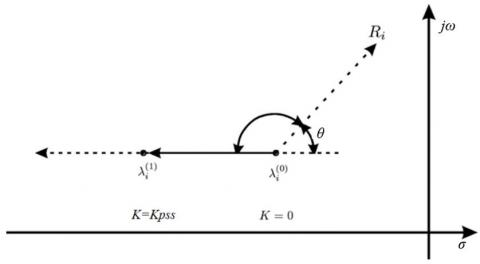Figure 1. Effect of displacement of mode by means of the residue 

3. Modelling of Power System Stabilizers with Feedback Control

The power system stabilizer is a device that is added to the excitation system of a synchronous generator, whose purpose is dampening the oscillations present in the electrical systems. Fulfill this purpose, and the PSS introduces an electric torque component in phase with the variation of the rotor speed [1, 2, 5].

As shown in Figure 2 indicates the stabilizer and parameters used for the study and adequate modeled using the DIgSILENT simulation language (DSL). The gain block (Kpss) allows amplified the input signal. The washout filter (Tw) allows passing the input signal components with frequency range for modes oscillations in general 0.1 Hz to 3.0 Hz. While that other filters called phase compensation blocks are used to modify the residue angle of selection mode oscillation how it indicates in the Eq. (11). The input signal to AVR has an appropriate limit all this allows defining a realistic value.

## 2.jpg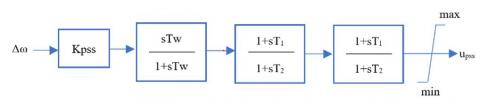Figure 2. Model power system stabilizer

where, KPSS and the time constant are tuning for methodology to exception of Tw is the time constant of the washout filter set in 10 seconds.

3.1 Tuning of power system stabilizers

The modification of parameters of PSS has the aim of tuning and moves the real part of λi to the left, as shown in Figure 1 .

In the following equations it shows the determination of the parameters of PSS (Kpss, T1, and T2) through residue of mode is used [5, 8, 9]:

$\left|\Delta \lambda_{i}\right|=\left|\sigma_{i}-\sigma_{\text {selec }}\right|$                       (12)

$K_{P S S}=\frac{\left|\Delta \lambda_{i}\right|}{\left|R_{i}\right|\left|H_{P S S}\right|}$                              (13)

$T_{1}=\frac{\sqrt{1+\sin \left(\frac{180^{o}-\theta}{2}\right)}}{w_{i} \sqrt{1-\sin \left(\frac{180^{o}-\theta}{2}\right)}}$              (14)

$T_{2}=\frac{1-\sin \left(\frac{180^{o}-\theta}{2}\right)}{1+\sin \left(\frac{180^{o}-\theta}{2}\right)} \cdot T_{1}$                (15)

3.2 Feedback of states control

The idea by including the control loop with the state variables (e.g., speed and voltage in electric power system) of the system is called state feedback allows the dynamic response of a system modify without the need to change any component of the system. This loop has advantages such as fast response time, better stability, less sensitivity to variations in system parameters [3, 10]. The control law is, in general, a second-order polynomial is used for systems with differential-algebraic variables where the dynamics of the system can be represented employing the Eq. (16) for the input signal u(t) and the output x(t).

$K u=\ddot{x}+a \dot{x}+b x$                   (16)

Since Eq. (16) is a differential equation that has two states, so the control law for the input variable must be like Eq. (17) since the first derivative depends on the output and the second depends on the first derivative .

$U=K_{1} \dot{x}+K_{2} x$                          (17)

3.3 Artificial neural network for tuning PSS

The characteristic of artificial neural networks (ANN) is the ability to learn a different type of output with different input without the need to know the relationships between input and output explicitly. The situation that can be used to determine the tuning parameters of the PSS considering the feedback on states of control presented for the Eq. (17).

The ANN is building with ntool of MATLAB® where learning algorithm used is backpropagation, this being the mechanism through which all the parameters of the network are adapted and modified, its operation is based on updates them in supervised learning process by the steepest descent gradient to minimize the mean square error through the adjustment of the weights [7-11]. A network neural established for each tuning parameter of the PSS.

4. Proposed Methodology

We are proposed to design the state feedback control requires access to the variable to be controlled and its reference, in the case of the AVR the control law to be implemented it is in the voltage regulators of the selected generators is presented in Eq. (18) for a power system.

$U=K_{1} V^{.}_{e r r}+K_{2} V_{e r r}$        (18)

The state feedback control model has implemented in DIgSILENT PowerFactory with its respective dynamic response. This work has been done in Digital Simulation Language (DSL) language and based on the Eq. (18). It shows in Figure 3. In this implementation, where the state feedback control law is established by feeding, on the one hand, the error signal constituted by the PSS signal the generator signals such as terminal voltage and the reference voltage. The other signal formed by those derived from the last signals.

## 3.jpgFigure 3. Model feedback control

For the determination of the parameters of each PSS selected, two independent ANNs. The first neural network has allowed calculating time constants T1, T2, and the second neural network determines the value of the gains of power system stabilizers. For each neural network, a set of variables over the electrical operation system was chosen as inputs. Active and reactive power of each generator dispatch is select.  Figure 4 shows the structure general of the neural network get used to obtaining T1, T2, and gain PSS of blocks show in Figure 2.

## 4.jpgFigure 4. Structure of ANN to determine the parameters of time and PSS gain respectively

Figure 5 shows a flow chart of the whole procedure that, once adequate modeling, the electrical system in DIgSILENT PowerFactory, which calculates five files, system matrices, eigenvalues, right eigenvector, left eigenvector, state matrix export to MATLAB here, in stage one a first script implemented with Eq. (10) To (15) reads and processes, these files estimate the matrix B and C, which finally used to get module and angle of the residue and calculate tuning of PSS. In the second stage, a second script implemented for determining the same parameters of tuning of PSS through ANN.

## 5.jpgFigure 5. Flow chart of proposed methodology

Finally, by analyzing the performance on the damping of low-frequency oscillations of SEP, it is a dynamic analysis is executed considering an event and parameters of PSSs in the system which is obtained through PowerFactory.

5. Simulation Results

In this section is presented the location of PSS by residue and tuning of parameters with control state feedback thus increasing the damping of the mode selected to the damping ratio desire, with that it gets a better grade of stability of power system in the PSS of generators selected by the residue method. In both systems.

The simulation of electrical systems was carried out in PowerFactory version 15.1.7, which incorporates studies of power flow, small-signal stability, time-domain simulations, among others, which together with MATLAB analysis tools 2017a version, allow to carry out the different steps of the methodology of section 4.

The first test system is the New England system formed with 10 generators and 39 buses and load with constant power was tested, as shown in Figure 6 [12, 13].

While the other test system corresponds to a real hydrothermal system of the Ecuadorian system, called the National Interconnected System. In 2018, it introduced a maximum demand of 23900.7 GWh of which 82.16% was produced by hydroelectric plants, 1.49% from renewable energy sources, 0.42% from interconnections with Colombia and Perú, 15.93% of the energy that could meet the demand for 2018 was obtained from 93 thermal generation units based on turbo steam, gas, diesel, and internal combustion engines, 27 hydraulic generation units and 5 renewable generation units with power generation installed of 7253 MW and 3199 km of 230 kV transmission lines in Figure 7 .

## 6.jpg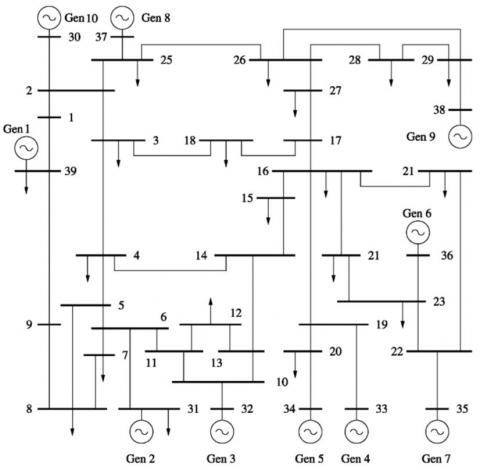Figure 6. Test power system New England of 39 bus

For the New England system, the modal analysis identified the oscillation modes less to limit the damping ratio than previously specified in this case is 5%. Table 1 presents the modes for the scenario without PSS. The results indicate that there are two oscillatory modes (73 and 91) with their respective conjugate complex mode situation that required the activation of PSS in the generators with the highest residues that allows controllability and observability of each mode.

Figure 8 shows the residue of mode 73 and mode 91, respectively. These indicate which generator's PSS must be activated. In the case of mode 73, generator G09 has the highest residue magnitude. Now by mode 91, the generator that has high residue is G05. Additionally, this situation indicates that in these generators must ubicate PSS with their feedback control.

At this moment is necessary tuning parameters for the activated PSS with the residue method determination of the time constants T1, T2, and gain of the model analyzed of PSS.

Another form to obtain the parameters of PSS without prior calculation of the residue method, it used the application of ANN, this allows determining the values of tuning the constant time and gain of the power system stabilizers of the generators selected through the module of the residue of the power systems studied.

Table 1. Modal analysis for New England System without PSS

 Mode Real  [1/s] Imaginary [rad/s] Angle [deg] Damping Frequency [Hz] Damping Ratio 00073 -0.078 6.428 90.692 1.023 0.012 00074 -0.078 -6.428 -90.692 1.023 0.012 00091 -0.168 4.111 92.335 0.654 0.041 00092 -0.168 -4.111 -92.335 0.654 0.041 00063 -0.345 6.685 92.956 1.064 0.052 00064 -0.345 -6.685 -92.956 1.064 0.052 00059 -0.399 7.386 93.092 1.176 0.054 00060 -0.399 -7.386 -93.092 1.176 0.054

## 7.jpgFigure 7. National interconnect system of Ecuador

## 8.jpgFigure 8. Residue for oscillation mode of test system

The architecture implemented of the neural network considered four layers, selecting the one that minimizes the mean square error, without affecting the stability of the results. That the input layer corresponds to the handling of the variables of the generators in which the PSS is located and activated, the second layer corresponds to 70% and the third layer 30% of the double placed in the first layer and the fourth layer set by the number of output variables that need to be determined.

For the case of time constants, two times the number of generators where the PSS is activated and for PSS gain the number of generators. The activation functions of the hidden and output layers were hyperbolic tangent in order to eliminate the out-of-range values of the variables.

Figure 9 represents the behavior of the neural network for training, validation, and testing. It established that the number of epochs required to minimize the error is proximity to 500 and showed to be adequate for the determination of the parameters of the PSS through the system operation variables without the need to perform the modal analysis that demands the determination of the state matrices and the eigenvalues and eigenvectors.

## 9.jpg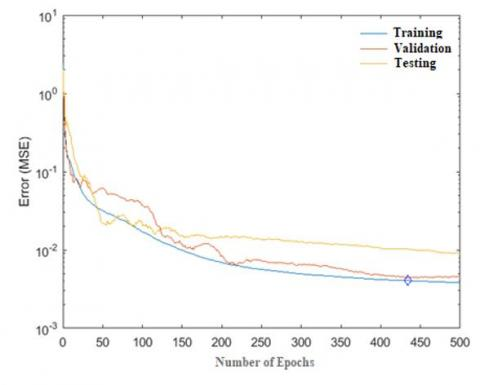Figure 9. Behavior of ANN for tune of parameters

Table 2 shows the comparison into the values obtained by the residue method and ANN are similarly.

Table 2. Comparison tuning parameters of PSS of New England system

 Name Residue ANN K T1 T2 K T1 T2 PSS-10 10.0 0.327 0.074 9.65 0.337 0.073 PSS-09 26.9 0.365 0.066 26.87 0.366 0.067 PSS-06 10.0 0.368 0.066 9.44 0.367 0.065 PSS-05 10.0 0.356 0.068 9.99 0.357 0.068

To demonstrate the tuning of parameters obtained for PSS, though, it uses of ANN and residue they are adequate. A system has been exposed to a contingency, to excite the oscillatory modes, in such a way exposing the generators to respond in the most critical scenario in the domain of time. The contingency selected consists of a three-phase short circuit of 0.1 seconds with the opening of the transmission line of bus 16-19.

Figure 10 shows the active power of each generator where it was activated PSS and parameters are some similarities with both methods. In all cases, see damping oscillations with that the response determined the stability of the system. This damping with PSS and feedback control shows a lower oscillation to reach a new point of stability.

Table 3 presents a modal analysis for each scenario with and without PSS whose parameters obtained for the ANN and residue method included or not feedback control. It concluded that the results obtained from ANN are similar because the tuning parameters with both methods determine a similar point of stability, all these allow reaching the damping desire for the test system was over 5%.

The second test system that is applied methodology is a real electric system of Ecuador. Location and tuning of PSS require information of oscillation modes that do not meet a level of the damping factor. An adequate damping factor looks for guarantees the stability of the system; for this system, it is considered 5% to ensure adequate damping of the oscillations as stated in the references [13, 14]. Therefore, the oscillation modes of interest correspond to those with a damping factor below the set level. For the scenario of maximum demand, the system state matrices are obtained, which allow the calculation of the residue. Table 4 contains the oscillation modes with the lowest damping factor of the scenario without considering the PSS.

## 10.jpg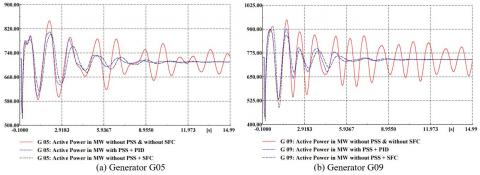Figure 10. Active power G05 and G09 of New England System

Table 3. Modal analysis for test New England system by scenario

 Scenario Mode Real [1/s] Imaginary [rad/s] Damping Frequency [Hz] Damping Ratio Without PSS 00073 -0.078 6.428 1.023 0.012 00074 -0.078 -6.428 1.023 0.012 00091 -0.168 4.111 0.654 0.041 00092 -0.168 -4.111 0.654 0.041 Residue With PSS Without Feedback Control 00065 -0.502 7.771 1.237 0.064 00066 -0.502 -7.771 1.237 0.064 00071 -0.479 6.661 1.060 0.072 00072 -0.479 -6.661 1.060 0.072 With PSS With Feedback Control 00065 -0.501 7.771 1.237 0.064 00066 -0.501 -7.771 1.237 0.064 00071 -0.481 6.677 1.063 0.072 00072 -0.481 -6.677 1.063 0.072 ANN With PSS Without Feedback Control 00065 -0.502 7.771 1.237 0.064 00066 -0.502 -7.771 1.237 0.064 00071 -0.470 6.662 1.060 0.070 00072 -0.470 -6.662 1.060 0.070 With PSS With Feedback Control 00065 -0.500 7.771 1.237 0.064 00066 -0.500 -7.771 1.237 0.064 00071 -0.460 6.676 1.063 0.069 00072 -0.460 -6.676 1.063 0.069

Table 4. Modal analysis for national interconnected system of Ecuador without PSS

 Mode Real  [1/s] Imaginary [rad/s] Angle [deg] Damping Frequency [Hz] Damping Ratio 00341 -0.234 7.262 91.847 1.156 0.032 00342 -0.234 -7.262 -91.847 1.156 0.032 00279 -0.517 11.374 92.601 1.810 0.045 00280 -0.517 -11.374 -92.601 1.810 0.045 00285 -0.517 10.643 92.782 1.694 0.049 00286 -0.517 -10.643 -92.782 1.694 0.049 00343 -0.439 8.626 92.915 1.373 0.051 00344 -0.439 -8.626 -92.915 1.373 0.051

The location and activation of PSSs are calculating the residue of the oscillation modes of interest in Figure 11 the residue of the oscillation modes 341, 279 and 285 are shown with a damping factor less than 5 %. The high residues are presented in 50, 60, 89, and 90 generators that represent three hydroelectric units of the system.

## 11.jpg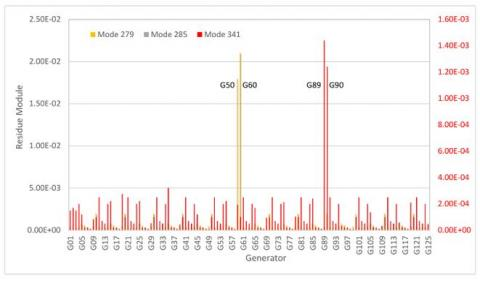Figure 11. Residue of national interconnected system of Ecuador

Through the equations, it is possible to determine the parameters of the PSSs of selected generators. Table 5 it can observe that parameters determined through ANN and residue are similar they are showing an adequate neural network behavior and potential by obtaining tuned of PSS since calculus of residue is computational high in real systems.

Table 5. Comparison tuning parameters PSS national interconnected system of Ecuador

 Name Residue ANN K T1 T2 K T1 T2 PSS G50/60 70.1 0.315 0.060 73.7 0.322 0.069 PSS G89 10.0 0.134 0.066 15.7 0.186 0.082 PSS G90 20.0 0.132 0.059 22.5 0.139 0.063
Time-domain simulations will be allowed to verify the effectiveness of location and tuning of PSSs on generator output power. Figure 12 shows the dynamic responses of the G50 and G89 hydroelectric generator of the multi-machine National Interconnected System of Ecuador has been performed by setting three-phase fault with the opening of the transmission line of Sto. Domingo-Quevedo to 0.1 seconds. Damping with PSS and feedback control shows the effectiveness of reducing oscillations to achieve system stability in less time than with conventional control elements.

Table 6 shows the modes of low-frequency oscillation. Small signal stability studies by modal analysis look for the damping ratio, frequency, and dominant oscillation mode through which of analysis it does with and without PSS its parameters are obtained by residue method included or not feedback control and so it does with ANN. It is concluded that network ANN allows tuning parameters and increases the point of stability, all modes allow it to reach the damping ratio of over 5%.

When the PSS and other control do not consider in the system, this shows oscillations that non-damped. These oscillations are damped, for example, with set PSS & PID, like to considered in article , with less amplitude and in a long time compared which the control SFC utilizes, where got better performance of the damping of the oscillations observed by reducing the amplitude from first oscillations. All this allows the system to reaches quickly and stronger stability when presents small-signal stability.

Table 6. Modal analysis for national interconnected system of Ecuador

 Scenario Mode Real [1/s] Imaginary [rad/s] Damping Frequency [Hz] Damping Ratio Without PSS 00341 -0.234 7.262 1.156 0.032 00279 -0.517 11.374 1.810 0.045 00285 -0.517 10.643 1.694 0.049 Residue With PSS Without Feedback Control 00357 -0.497 7.035 1.120 0.070 00359 -0.532 7.453 1.186 0.071 00150 -1.302 19.572 3.115 0.066 With PSS With Feedback Control 00384 -0.369 6.269 0.998 0.059 00166 -1.302 19.571 3.115 0.066 00378 -0.492 7.035 1.120 0.070 ANN With PSS Without Feedback Control 00147 -1.303 19.585 3.117 0.067 00356 -0.500 7.076 1.126 0.071 00350 -0.455 6.349 1.010 0.071 With PSS With Feedback Control 00351 -0.383 6.298 1.002 0.065 00166 -1.302 19.57 3.115 0.068 00357 -0.493 7.041 1.121 0.072

## 12.jpg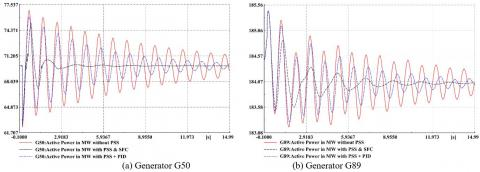Figure 12. Active power G50 and G89 of national interconnected system of Ecuador

6. Conclusions

In this study, it was able to illustrate the capacity the residue method allows to determine the generation units in which damping oscillations locate the stabilizers in the system power with inclusion the control loop for state feedback additionally is shown an ANN for tuning parameters of PSS.

Through both electrical systems present in this study. First, the test system which models the characteristics of an overall electrical system where a 5% damping factor for the oscillation modes generally used by system operators was proposed, through which to ensure robust stability before contingencies. These restrictions were reached more quickly and robustly by the proposed control method. These positive results justify the study carried out and constitute the starting point to continue with the analysis of other more complex control methods to apply them to electric power systems.

The proposed approach shows its potential for determining the generators of each scenario where the relevant low-frequency modes are determined, and the PSS performance improves its effectiveness by including an additional control element, as seen in simulations in the time domain.

Nomenclature
 Δx The state vector of dimension n state. Δy The output vector of dimension m output. Δu The input vector of dimension r input. ANN Artificial Neural Network AVR Automatic Voltage Regulator A The n by n state matrix. B The n by r input matrix. C The m by n output matrix. D The m by r feed forward matrix. λ Eigenvalues. σ Real part of eigenvalues. ω Imaginary part eigenvalues and frequency of the researched mode of oscillation [rad/sec] ξ Damping of mode of oscillation. ϕ Right eigenvector. ψ Left eigenvector. HPSS Function of feedback control. KPSS Gain of PSS PSS Power System Stabilizer SFC State Feedback Control MSE Mean square error T1 Time constant of led-lag filter [s]. T2 Time constant of led-lag filter [s]. Tw Time constant of washout filter [s]. θ Phase angle of the residue Ri [rad] Δλi Variation of the mode to be damped.
References

 Pota, H.R. (2018). The essentials of power system dynamics and control. Springer Nature Singapore Pte Ltd. https://doi.org/10.1007/978-981-10-8914-5

 Kundur, P., Balu, N.J., Lauby, M.G. (1994). Power System Stability and Control. New York: McGraw-hill.

 Pal, B., Chaudhuri, B. (2006). Robust Control in Power Systems. Springer Science & Business Media.

 Su, C., Hu, W., Fang, J., Chen, Z. (2013). Residue-based coordinated selection and parameter design of multiple power system stabilizers (PSSs). In IECON 2013-39th Annual Conference of the IEEE Industrial Electronics Society, Vienna, Austria. https://doi.org/10.1109/IECON.2013.6699465

 Li, Y., Rehtanz, C., Ruberg, S., Luo, L., Cao, Y. (2012). Assessment and choice of input signals for multiple HVDC and FACTS wide-area damping controllers. IEEE Transactions on Power Systems, 27(4): 1969-1977. https://doi.org/10.1109/TPWRS.2012.2189865

 Chen, X., Li, X., Ren, Z., Zheng, T. (2018). Wide-area feedback signal and control location selection for low-frequency oscillation. AIMEE2018 2018: Advances in Intelligent Systems and Computing, 902: 759-769. https://doi.org/10.1007/978-3-030-12082-5_69

 Lai, L.L. (1998). Intelligent System Applications in Power Engineering: Evolutionary Programming and Neural Networks. John Wiley & Sons, Inc.

 Germanos, M. (2015) Power System Stability Response and Control Using Small Signal Analysis, Master thesis, University of Boston, Massachusetts, USA.

 Hannan, M., Islam, N.N., Mohamed, A., Molla S. Lipu, H., Ker, P.J., Rashid, M.M., Shareef, H. (2018). Artificial intelligent-based damping controller optimization for the multi-machine power system a review. IEEE Access, (6): 39574-39594. https://doi.org/10.1109/ACCESS.2018.2855681

 Dussaud, F. (2015) An application of modal analysis in electric power systems to study inter-area oscillations. Master Thesis, KTH Royal Institute of Technology School of Electrical Engineering, Sweden.

 Yeu, H. (2010). Small signal analysis of power systems: eigenvalue tracking method and eigenvalue estimation contingency screening for DSA. Ph.D. dissertation, University of Illinois, Urbana-Champaign, USA.

 Flores, H.B., Cepeda, J.C., Gallardo, C.F. (2017). Optimum location and tuning of PSS devices considering multi-machine criteria and a heuristic optimization algorithm. In 2017 IEEE PES Innovative Smart Grid Technologies Conference-Latin America (ISGT Latin America), pp. 1-6. https://doi.org/10.1109/ISGT-LA.2017.8126712

 Surinkaew, T., Ngamroo, I. (2018). Adaptive signal selection of wide-area damping controllers under various operating conditions. IEEE Transactions on Industrial Informatics, 14(2): 639-651. https://doi.org/10.1109/TII.2017.2752762

 Ecuador ISO-CENACE. (2019). Annual Report 2018. CENACE, Quito.

 Derrar, A., Nacer, A. (2017). Particle swarm optimization for tuning PSS-PID controller of synchronous generator. Journal of Automation, Mobile Robotics & Intelligent Systems, 11(1): 48-52. https://doi.org/10.14313/JAMRIS_1-2017/6